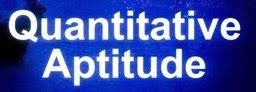Quant Quiz for IBPS Clerk Mains, India Post

Directions (Q. 1 - 5): In each of the following questions, a series of numbers which contains a wrong number is given. Find out the wrong number in each case.1. 1, 6, 13, 24, 33, 46, 61
1) 6
2) 13
3) 24
4) 33
5) 46

2. 8, 8, 9, 9, 11, 10, 13, 11, 18
1) 8
2) 9
3) 10
4) 11
5) 13

3. 3, 5, 13, 43, 175, 891, 5353
1) 43
2) 175
3) 891
4) 5353
5) None of these

4. 5, 10, 10, 10, 15, 10, 40, 10, 80
1) 5
2) 10
3) 15
4) 40
5) 80

5. 6, 9, 15, 19, 29, 47, 81
1) 9
2) 15
3) 19
4) 29
5) 47

6. An article was sold for certain price and there was a gain of 50%. If it would have been sold for Rs. 80 Less, there would be neither gain nor loss. Find the C.P.?
1) Rs. 100
2) Rs. 130
3) Rs. 160
4) Rs. 150
5) Can't be determined

7. A man is walking around a rectangular park at a speed of 7 km/h. and completes one round in 20 minute. What is the perimeter of the park?
1) 110 km.
2) 140 m.
3) 2.33 km.
4) 1.35 km.
5) 110 m.

8. In how may ways 'RECEPTION' can be written so that vowel and consonant come alternately?
1) 120
2) 60
3) 420
4) 1400
5) 2880

9. The perimeter of the square is same that of a rectangle. If side of square is 14 m, what is the length of rectangle?
1) 14 m.
2) 28 m.
3) 7 m.
4) 14 cm.
5) Can 't be determined

10. The radius of a circular garden is 13 m. there is a path 2 m. wide outside it. Find the area of the path outside-
1) 707 m^2
2) 531 m^2
3) 100 m^2
4) 176 m^2
5) 150 m^2

1. 3

1 + 5 = 6, 6 + 7 = 13, 13 + 9 = 22, ...

2. 5

There are two series,
S1 = 8 + 1 = 9, 9 + 2 = 11, 11 + 3 = 14, ...
S2 = 8 + 1 = 9, 9 + 1 = 10, 10 + 1 = 11, ...

3. 2

The series is:
3 × 1 + 2 = 5
5 × 2 + 3 = 13
13 × 3 + 4 = 43
43 × 4 + 5 = 177
177 × 5 + 6 = 891
891 × 6 + 7 = 5353

4. 3

There are two series:
S1 = 5 × 2 = 10, 10 × 2 = 20, 20 × 2 = 40 ...
S2 = 10 + 0 = 10, 10 + 0 = 10, 10 + 0 ...

5. 2

6 × 2 - 3 = 9, 9 × 2 - 5 = 13,
13 × 2 - 7 = 19, ...

6. 3

Let CP = x

SP = y

So, (y - x)/x * 100 = 50
y = 3x/2
3x/2 - 80 = x
x = 160

7. 3; Distance travelled = Perimeter

8. 5; 5 consonant + 4 vowel  5! × 4!

9. 5;

10. 4;